•#### Learning Bayesian Models with R#### Overview of this book

Learning Bayesian Models with RCreditswww.PacktPub.comPrefaceFree Chapter
Introducing the Probability TheoryThe R EnvironmentIntroducing Bayesian InferenceMachine Learning Using Bayesian InferenceBayesian Regression ModelsBayesian Classification ModelsBayesian Models for Unsupervised LearningBayesian Neural NetworksBayesian Modeling at Big Data ScaleIndex## Exercises

1. By using the definition of conditional probability, show that any multivariate joint distribution of N random variables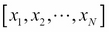has the following trivial factorization: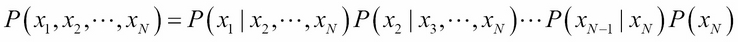2. The bivariate normal distribution is given by: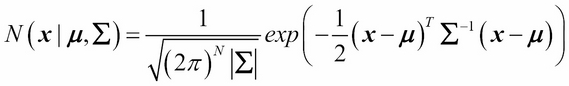Here: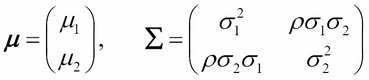By using the definition of conditional probability, show that the conditional distribution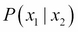can be written as a normal distribution of the form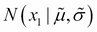where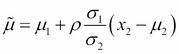and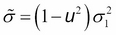.

3. By using explicit integration of the expression in exercise 2, show that the marginalization of bivariate normal distribution will result in univariate normal distribution.

4. In the following table, a dataset containing the measurements of petal and sepal sizes of 15 different Iris flowers are shown (taken from the Iris dataset, UCI machine learning dataset repository). All units are in cms:

Sepal Length

Sepal Width

Petal Length

Petal Width

Class of Flower

5.1

3.5

1.4

0.2

Iris-setosa

4.9

3

1.4

0.2

Iris-setosa

4.7

3.2

1.3

0.2

Iris-setosa

4.6

3.1

1.5

0.2

Iris-setosa

5

3.6

1.4

0.2

Iris-setosa

7

3.2

4.7

1.4

Iris-versicolor

6.4

3.2

4.5

1.5

Iris-versicolor

6.9

3.1

4.9

1.5

Iris-versicolor

5.5

2.3

4

1.3

Iris-versicolor

6.5

2.8

4.6

1.5

Iris-versicolor

6.3

3.3

6

2.5

Iris-virginica

5.8

2.7

5.1

1.9

Iris-virginica

7.1

3

5.9

2.1

Iris-virginica

6.3

2.9

5.6

1.8

Iris-virginica

6.5

3

5.8

2.2

Iris-virginica